# The rough graph of the function y = 1 − 1 2 e − x use transformations if needed.### Single Variable Calculus: Concepts...

4th Edition
James Stewart
Publisher: Cengage Learning
ISBN: 9781337687805### Single Variable Calculus: Concepts...

4th Edition
James Stewart
Publisher: Cengage Learning
ISBN: 9781337687805

#### Solutions

Chapter 1.5, Problem 15E
To determine

## To sketch: The rough graph of the function y=1−12e−x use transformations if needed.

Expert Solution

### Explanation of Solution

The standard graph of the function y=ex is roughly sketched as shown below in Figure 1.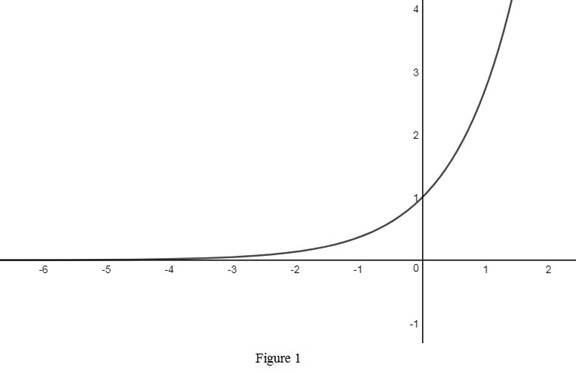Reflect the graph y=ex about the y-axis and obtain the graph of y=ex . Thus, the graph of y=ex is drawn and shown below in Figure 2.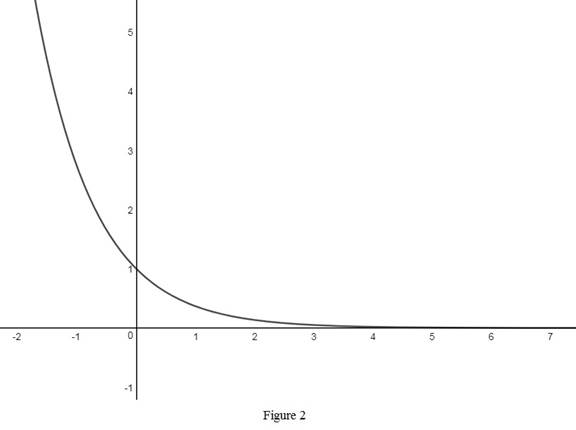Then, to draw the graph of y=ex , reflect the graph y=ex about the x-axis. Thus, the graph of y=ex is shown below in Figure 3.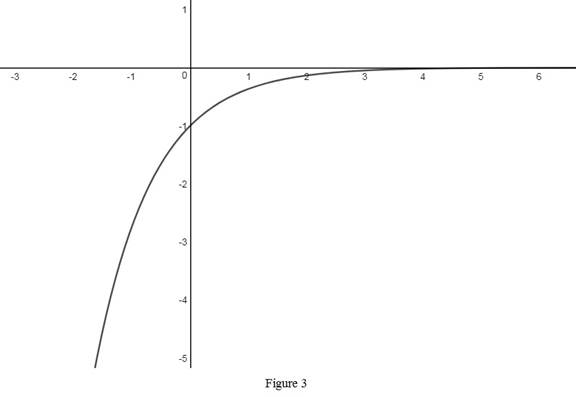Then, draw the graph of y=12ex , by shrunk the graph y=ex vertically by a factor of 0.5. Thus, the graph of y=12ex is shown below in Figure 4.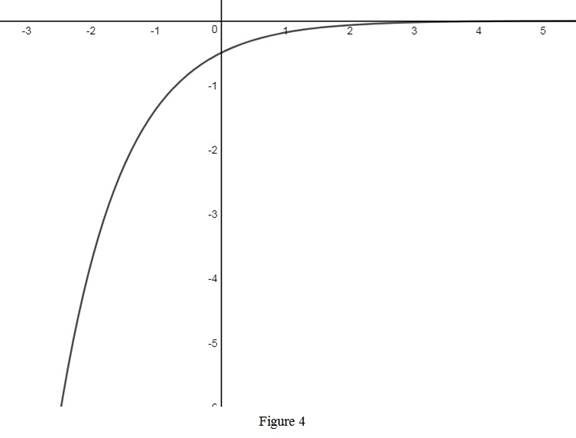Then, to obtain the graph of y=112ex , shift the graph y=12ex one unit upward. Thus, the graph of y=112ex is shown below in Figure 5.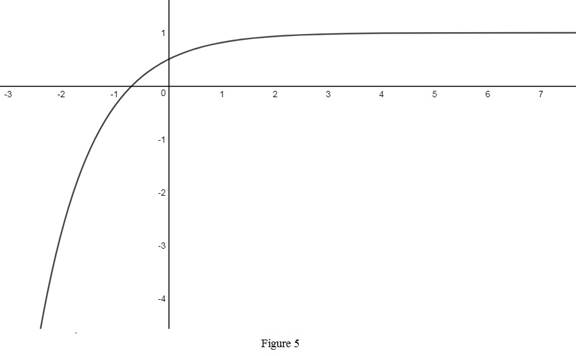Thus, the required graph y=112ex is obtained from the basic graph y=ex by performing some transformations.

### Have a homework question?

Subscribe to bartleby learn! Ask subject matter experts 30 homework questions each month. Plus, you’ll have access to millions of step-by-step textbook answers!